Most Affordable JEE | NEET | 8,9,10 Preparation by Kota's Top IITian Doctor Faculties

# NCERT Solutions for Class 11 Physics chapter 9 - Mechanical Properties of solid - Free PDF downloadHey, are you a class 11 student and looking for ways to download NCERT Solutions for Class 11 Physics chapter 9 "Mechanical Properties of solid"? If yes. Then read this post till the end.

In this article, we have listed NCERT solution for class 11 Physics Chapter 9 in PDF that are prepared by Kota’s top IITian’s Faculties by keeping Simplicity in mind.

If you want to learn and understand class 11 Physics chapter 9 "Mechanical Properties of solid" in an easy way then you can use these solutions PDF.

NCERT Solutions helps students to Practice important concepts of subjects easily. Class 11 Physics solutions provide detailed explanations of all the NCERT questions that students can use to clear their doubts instantly.

If you want to score high in your class 11 Physics Exam then it is very important for you to have a good knowledge of all the important topics, so to learn and practice those topics you can use eSaral NCERT Solutions.

So, without wasting more time Let’s start.

### Download The PDF of NCERT Solutions for Class 11 Physics Chapter 9 "Mechanical Properties of solid"

Question $1$ : A steel wire of length $4.7 \mathrm{~m}$ and cross-sectional area $3.0 \times 10^{-5} \mathrm{~m}^{2}$ stretches by the same amount as a copper wire of length $3.5 \mathrm{~m}$ and cross-sectional area of $4.0 \times 10^{-5} \mathrm{~m}^{2}$ under a given load. What is the ratio of the Young's modulus of steel to that of copper?

Solution. Length of the steel wire, $L_{1}=4.7 \mathrm{~m}$
Area of cross-section of the steel wire, $A_{1}=3.0 \times 10^{-5} \mathrm{~m}^{2}$
Length of the copper wire, $L_{2}=3.5 \mathrm{~m}$
Area of cross-section of the copper wire, $A_{2}=4.0 \times 10^{-5} \mathrm{~m}^{2}$
Change in length $=\Delta L_{1}=\Delta L_{2}=\Delta L$
Force applied in both the cases $=F$
Young's modulus of the steel wire:
$Y_{1}=\frac{F_{1}}{A_{1}} \times \frac{L_{1}}{\Delta L}$
$=\frac{F \times 4.7}{3.0 \times 10^{-5} \times \Delta L} \ldots(i)$
Young’s modulus of the copper wire:
\begin{aligned} Y_{2} &=\frac{F_{2}}{A_{2}} \times \frac{L_{2}}{\Delta L_{2}} \\ &=\frac{F \times 3.5}{4.0 \times 10^{-5} \times \Delta L} \end{aligned}
\begin{aligned} Y_{2} &=\frac{F_{2}}{A_{2}} \times \frac{L_{2}}{\Delta L_{2}} \\ &=\frac{F \times 3.5}{4.0 \times 10^{-5} \times \Delta L} \end{aligned}$$\ldots(i i) Dividing (i) by (ii), we get: \frac{Y_{1}}{Y_{2}}=\frac{4.7 \times 4.0 \times 10^{-5}}{3.0 \times 10^{-5} \times 3.5}=1.79: 1 The ratio of Young’s modulus of steel to that of copper is 1.79 : 1. Question 2: Figure 9.11 shows the strain-stress curve for a given material. What are (a) Young’s modulus and (b) approximate yield strength for this material?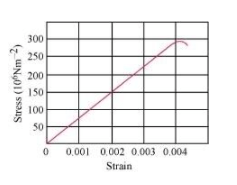Solution. (a) It is clear from the given graph that for stress 150 \times 10^{6} \mathrm{~N} / \mathrm{m}^{2}, strain is 0.002 \therefore Young's modulus, Y=\frac{\text { Stress }}{\text { Strain }} =\frac{150 \times 10^{6}}{0.002}=7.5 \times 10^{10} \mathrm{~N} / \mathrm{m}^{2} Hence, Young's modulus for the given material is 7.5 \times 10^{10} \mathrm{~N} / \mathrm{m}^{2}. (b) The yield strength of a material is the maximum stress that the material can sustain without crossing the elastic limit. It is clear from the given graph that the approximate yield strength of this material is 300 \times 10^{6} \mathrm{Nm} /{ }^{2} or 3 \times 10^{8} \mathrm{~N} / \mathrm{m}^{2}. Question 3: The stress-strain graphs for materials A and B are shown in Fig. 9.12.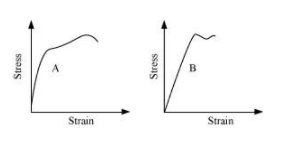The graphs are drawn to the same scale. (a) Which of the materials has the greater Young’s modulus? (b) Which of the two is the stronger material? Solution(a) A (b) A (a) For a given strain, the stress for material A is more than it is for material B, as shown in the two graphs. Young’s modulus=\frac{\text { Stress }}{\text { Strain }} For a given strain, if the stress for a material is more, then Young’s modulus is also greater for that material. Therefore, Young’s modulus for material A is greater than it is for material B. (b) The amount of stress required for fracturing a material, corresponding to its fracture point, gives the strength of that material. Fracture point is the extreme point in a stress-strain curve. It can be observed that material A can withstand more strain than material B. Hence, material A is stronger than material B. Question 4: Read the following two statements below carefully and state, with reasons, if it is true or false. (a) The Young’s modulus of rubber is greater than that of steel; (b) The stretching of a coil is determined by its shear modulus. Solution. Solution. (a) False (b) True (a) For a given stress, the strain in rubber is more than it is in steel. Young’s modulus, Y=\frac{\text { Stress }}{\text { Strain }} For a constant stress: Y \propto \frac{1}{\text { Strain }} Hence, Young’s modulus for rubber is less than it is for steel. (b) Shear modulus is the ratio of the applied stress to the change in the shape of a body. The stretching of a coil changes its shape. Hence, shear modulus of elasticity is involved in this process. Question 5: Two wires of diameter 0.25 cm, one made of steel and the other made of brass are loaded as shown in Fig. 9.13. The unloaded length of steel wire is 1.5 m and that of brass wire is 1.0 m. Compute the elongations of the steel and the brass wires. Solution.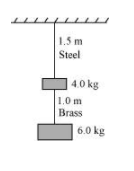Solution. Elongation of the steel wire =1.49 \times 10^{-4} \mathrm{~m} Elongation of the brass wire =1.3 \times 10^{-4} \mathrm{~m} Diameter of the wires, d=0.25 \mathrm{~m} Hence, the radius of the wires, r=\frac{d}{2}=0.125 \mathrm{~cm} Length of the steel wire, L_{1}=1.5 \mathrm{~m} Length of the brass wire, L_{2}=1.0 \mathrm{~m} Total force exerted on the steel wire: F_{1}=(4+6) \mathrm{g}=10 \times 9.8=98 \mathrm{~N} Young's modulus for steel: Y_{1}=\frac{\left(\frac{F_{1}}{A_{1}}\right)}{\left(\frac{\Delta L_{1}}{L_{1}}\right)} Where, \Delta L_{1}= Change in the length of the steel wire A_{1}= Area of cross-section of the steel wire =\pi r_{1}^{2} Young's modulus of steel, Y_{1}=2.0 \times 10^{11} \mathrm{~Pa} \therefore \Delta L_{1}=\frac{F_{1} \times L_{1}}{A_{1} \times Y_{1}}=\frac{F_{1} \times L_{1}}{\pi r_{1}^{2} \times Y_{1}} =\frac{98 \times 1.5}{\pi\left(0.125 \times 10^{-2}\right)^{2} \times 2 \times 10^{11}}=1.49 \times 10^{-4} \mathrm{~m} Total force on the brass wire: F_{2}=6 \times 9.8=58.8 \mathrm{~N} Young's modulus for brass: Y_{2}=\frac{\left(\frac{F_{2}}{A_{2}}\right)}{\left(\frac{\Delta L_{2}}{L_{2}}\right)} Where, \Delta L_{2}= Change in length A_{2}= Area of cross-section of the brass wire \therefore \Delta L_{2}=\frac{F_{2} \times L_{2}}{A_{2} \times Y_{2}}=\frac{F_{2} \times L_{2}}{\pi r_{2}^{2} \times Y_{2}} =\frac{58.8 \times 1.0}{\pi \times\left(0.125 \times 10^{-2}\right)^{2} \times\left(0.91 \times 10^{11}\right)}=1.3 \times 10^{-4} \mathrm{~m} Elongation of the steel wire =1.49 \times 10^{-4} \mathrm{~m} Elongation of the brass wire =1.3 \times 10^{-4} \mathrm{~m} Question 6: The edge of an aluminium cube is 10 \mathrm{~cm} long. One face of the cube is firmly fixed to a vertical wall. A mass of 100 \mathrm{~kg} is then attached to the opposite face of the cube. The shear modulus of aluminium is 25 \mathrm{GPa}. What is the vertical deflection of this face? Soution. Edge of the aluminium cube, L=10 \mathrm{~cm}=0.1 \mathrm{~m} The mass attached to the cube, m=100 \mathrm{~kg} Shear modulus (n) of aluminium =25 \mathrm{GPa}=25 \times 10^{9} \mathrm{~Pa} Shear modulus, \eta=\frac{\text { Shear stress }}{\text { Shear strain }}=\frac{\frac{F}{A}}{\frac{L}{\Delta L}} Where, F= Applied force =m g=100 \times 9.8=980 \mathrm{~N} A= Area of one of the faces of the cube =0.1 \times 0.1=0.01 \mathrm{~m}^{2} \Delta L= Vertical deflection of the cube \therefore \Delta L=\frac{F L}{A \eta} =\frac{980 \times 0.1}{10^{-2} \times\left(25 \times 10^{9}\right)} =3.92 \times 10^{-7} \mathrm{~m} The vertical deflection of this face of the cube is 3.92 \times 10^{-7} \mathrm{~m}. Question 7: Four identical hollow cylindrical columns of mild steel support a big structure of mass 50,000 \mathrm{~kg}. The inner and outer radii of each column are 30 \mathrm{~cm} and 60 \mathrm{~cm} respectively. Assuming the load distribution to be uniform, calculate the compressional strain of each column. Solution. Mass of the big structure, M=50,000 \mathrm{~kg} Inner radius of the column, r=30 \mathrm{~cm}=0.3 \mathrm{~m} Outer radius of the column, R=60 \mathrm{~cm}=0.6 \mathrm{~m} Young's modulus of steel, Y=2 \times 10^{11} \mathrm{~Pa} Total force exerted, F=M \mathrm{~g}=50000 \times 9.8 \mathrm{~N} Stress = Force exerted on a single column =\frac{50000 \times 9.8}{4}=122500 \mathrm{~N} Young's modulus, Y=\frac{\text { Stress }}{\text { Strain }} Strain =\frac{F}{A} Where, Area, A=\pi\left(R^{2}-r^{2}\right)=\pi\left((0.6)^{2}-(0.3)^{2}\right) Strain =\frac{122500}{\pi\left[(0.6)^{2}-(0.3)^{2}\right] \times 2 \times 10^{11}}=7.22 \times 10^{-7} Hence, the compressional strain of each column is 7.22 \times 10^{-7}. Question 8: A piece of copper having a rectangular cross-section of 15.2 \mathrm{~mm} \times 19.1 \mathrm{~mm} is pulled in tension with 44,500 \mathrm{~N} force, producing only elastic deformation. Calculate the resulting strain? Solution. Length of the piece of copper, 1=19.1 \mathrm{~mm}=19.1 \times 10^{-3} \mathrm{~m} Breadth of the piece of copper, b=15.2 \mathrm{~mm}=15.2 \times 10^{-3} \mathrm{~m} Area of the copper piece: A=/ \times b =19.1 \times 10^{-3} \times 15.2 \times 10^{-3} =2.9 \times 10^{-4} \mathrm{~m}^{2} Tension force applied on the piece of copper, F=44500 \mathrm{~N} Modulus of elasticity of copper, \eta=42 \times 10^{\circ} \mathrm{N} / \mathrm{m}^{2} Modulus of elasticity, \eta=\frac{\text { Stress }}{\text { Strain }}=\frac{\frac{F}{A}}{\text { Strain }} \therefore Strain =\frac{F}{A \eta} =\frac{44500}{2.9 \times 10^{-4} \times 42 \times 10^{9}} =3.65 \times 10^{-3} Question 9: A steel cable with a radius of 1.5 \mathrm{~cm} supports a chairlift at a ski area. If the maximum stress is not to exceed 10^{8} \mathrm{~N} \mathrm{~m}^{-2}, what is the maximum load the cable can support? Solution. Radius of the steel cable, r=1.5 \mathrm{~cm}=0.015 \mathrm{~m} Maximum allowable stress =10^{8} \mathrm{~N} \mathrm{~m}^{-2} Maximum stress =\frac{\text { Maximum force }}{\text { Area of cross-section }} \therefore Maximum force = Maximum stress \times Area of cross-section =10^{8} \times \pi(0.015)^{2} =7.065 \times 10^{4} \mathrm{~N} Hence, the cable can support the maximum load of 7.065 \times 10^{4} \mathrm{~N}. Question 10: A rigid bar of mass 15 \mathrm{~kg} is supported symmetrically by three wires each 2.0 \mathrm{~m} long. Those at each end are of copper and the middle one is of iron. Determine the ratio of their diameters if each is to have the same tension. Solution. The tension force acting on each wire is the same. Thus, the extension in each case is the same. Since the wires are of the same length, the strain will also be the same. The relation for Young’s modulus is given as: Y=\frac{\text { Stress }}{\text { Strain }}=\frac{\frac{F}{A}}{\text { Strain }}=\frac{\frac{4 F}{\pi d^{2}}}{\text { Strain }} Where, F= Tension force A= Area of cross-section d= Diameter of the wire It can be inferred from equation (i) that Y \propto \frac{1}{d^{2}} Young's modulus for iron, Y_{1}=190 \times 10^{9} \mathrm{~Pa} Diameter of the iron wire =d_{1} Young's modulus for copper, Y_{2}=120 \times 10^{9} \mathrm{~Pa} Diameter of the copper wire =d_{2} Therefore, the ratio of their diameters is given as: \frac{d_{2}}{d_{1}}=\sqrt{\frac{Y_{1}}{Y_{2}}}=\sqrt{\frac{190 \times 10^{9}}{120 \times 10^{9}}}=\sqrt{\frac{19}{12}}=1.25: 1 Question 11: A 14.5 \mathrm{~kg} mass, fastened to the end of a steel wire of unstretched length 1.0 \mathrm{~m}, is whirled in a vertical circle with an angular velocity of 2 rev/s at the bottom of the circle. The cross-sectional area of the wire is 0.065 \mathrm{~cm}^{2}. Calculate the elongation of the wire when the mass is at the lowest point of its path. Slution. Mass, m=14.5 \mathrm{~kg} Length of the steel wire, l=1.0 \mathrm{~m} Angular velocity, \omega=2 \mathrm{rev} / \mathrm{s}=2 \times 2 \pi \mathrm{rad} / \mathrm{s}=12.56 \mathrm{rad} / \mathrm{s} Cross-sectional area of the wire, a=0.065 \mathrm{~cm}^{2}=0.065 \times 10^{-4} \mathrm{~m}^{2} Let \Delta / be the elongation of the wire when the mass is at the lowest point of its path. When the mass is placed at the position of the vertical circle, the total force on the mass is: F=m g+m / \omega^{2} =14.5 \times 9.8+14.5 \times 1 \times(12.56)^{2} =2429.53 \mathrm{~N} Young's modulus =\frac{\text { Stress }}{\text { Strain }} Y=\frac{F}{\frac{A}{\Delta l}}{l}=\frac{F}{A} \frac{l}{\Delta l} \therefore \Delta l=\frac{F l}{A Y} Young's modulus for steel =2 \times 10^{11} \mathrm{~Pa} \Delta l=\frac{2429.53 \times 1}{0.065 \times 10^{-4} \times 2 \times 10^{11}} \Rightarrow \Delta l=1.87 \times 10^{-3} \mathrm{~m} Hence, the elongation of the wire is 1.87 \times 10^{-3} \mathrm{~m}. Question 12: Compute the bulk modulus of water from the following data: Initial volume =100.0 litre, Pressure increase =100.0 \mathrm{~atm}\left(1 \mathrm{~atm}=1.013 \times 10^{5} \mathrm{~Pa}\right), Final volume =100.5 litre. Compare the bulk modulus of water with that of air (at constant temperature). Explain in simple terms why the ratio is so large. Solution. Initial volume, V_{1}=100.0 \mathrm{l}=100.0 \times 10^{-3} \mathrm{~m}^{3} Final volume, V_{2}=100.5 \mathrm{l}=100.5 \times 10^{-3} \mathrm{~m}^{3} Increase in volume, \Delta V=V_{2}-V_{1}=0.5 \times 10^{-3} \mathrm{~m}^{3} Increase in pressure, \Delta 0 =100.0 \mathrm{~atm}=100 \times 1.013 \times 10^{5} \mathrm{~Pa} Bulk modulus =\frac{\Delta p}{\frac{\Delta V}{V_{1}}}=\frac{\Delta p \times V_{1}}{\Delta V} =\frac{100 \times 1.013 \times 10^{3} \times 100 \times 10^{-3}}{0.5 \times 10^{-3}} =2.026 \times 10^{9} \mathrm{~Pa} Bulk modulus of air =1.0 \times 10^{5} \mathrm{~Pa} \therefore \frac{\text { Bulk modulus of water }}{\text { Bulk modulus of air }}=\frac{2.026 \times 10^{9}}{1.0 \times 10^{5}}=2.026 \times 10^{4} This ratio is very high because air is more compressible than water. Question 13: What is the density of water at a depth where pressure is 80.0 atm, given that its density at the surface is 1.03 \times 10^{3} \mathrm{~kg} \mathrm{~m}^{-3} ? Solution. Let the given depth be h. Pressure at the given depth, p=80.0 \mathrm{~atm}=80 \times 1.01 \times 10^{5} \mathrm{~Pa} Density of water at the surface, \rho_{1}=1.03 \times 10^{3} \mathrm{~kg} \mathrm{~m}^{-3} Let \rho_{2} be the density of water at the depth h. Let V_{1} be the volume of water of mass m at the surface. Let V_{2} be the volume of water of mass m at the depth h. Let \Delta V be the change in volume. \Delta V=V_{1}-V_{2} =m\left(\frac{1}{\rho_{1}}-\frac{1}{\rho_{2}}\right) \therefore Volumetric strain =\frac{\Delta V}{V_{1}} =m\left(\frac{1}{\rho_{1}}-\frac{1}{\rho_{2}}\right) \times \frac{\rho_{1}}{m} \therefore \frac{\Delta V}{V_{1}}=1-\frac{\rho_{1}}{\rho_{2}}$$\ldots(i)
Bulk modulus, $B=\frac{p V_{1}}{\Delta V}$
$\frac{\Delta V}{V_{1}}=\frac{p}{B}$
Compressibity of water $=\frac{1}{B}=45.8 \times 10^{-11} \mathrm{~Pa}^{-1}$
$\therefore \frac{\Delta V}{V_{1}}=80 \times 1.013 \times 10^{5} \times 45.8 \times 10^{-11}=3.71 \times 10^{-3}$$\ldots(i i) For equations (i) and (ii), we get: 1-\frac{\rho_{1}}{\rho_{2}}=3.71 \times 10^{-3} \rho_{2}=\frac{1.03 \times 10^{3}}{1-\left(3.71 \times 10^{-3}\right)} =1.034 \times 10^{3} \mathrm{~kg} \mathrm{~m}^{-3} Therefore, the density of water at the given depth (h) is 1.034 \times 10^{3} \mathrm{~kg} \mathrm{~m}^{-3}. Question 14: Compute the fractional change in volume of a glass slab, when subjected to a hydraulic pressure of 10 atm. Solution Hydraulic pressure exerted on the glass slab, p=10 \mathrm{~atm}=10 \times 1.013 \times 10^{5} \mathrm{~Pa} Bulk modulus of glass, B=37 \times 10^{9} \mathrm{Nm}^{-2} Bulk modulus, B=\frac{p}{\frac{\Delta V}{V}}Where, \frac{\Delta V}{V}= Fractional change in volume \therefore \frac{\Delta V}{V}=\frac{p}{B} =\frac{10 \times 1.013 \times 10^{5}}{37 \times 10^{9}} =2.73 \times 10^{-5} Hence, the fractional change in the volume of the glass slab is 2.73 \times 10^{-5} Question 15: Determine the volume contraction of a solid copper cube, 10 \mathrm{~cm} on an edge, when subjected to a hydraulic pressure of 7.0 \times 10^{6} \mathrm{~Pa}. Solution. Length of an edge of the solid copper cube, l=10 \mathrm{~cm}=0.1 \mathrm{~m} Hydraulic pressure, p=7.0 \times 10^{6} \mathrm{~Pa} Bulk modulus of copper, B=140 \times 10^{9} \mathrm{~Pa} Bulk modulus, B=\frac{P}{\frac{\Delta V}{V}} Where, \frac{\Delta V}{V}= Volumetric strain \Delta V= Change in volume V= Original volume. \Delta V=\frac{p V}{B} Original volume of the cube, V=\beta \therefore \Delta V=\frac{p l^{3}}{B} =\frac{7 \times 10^{6} \times(0.1)^{3}}{140 \times 10^{9}} =5 \times 10^{-8} \mathrm{~m}^{3} =5 \times 10^{-2} \mathrm{~cm}^{-3} Therefore, the volume contraction of the solid copper cube is 5 \times 10^{-2} \mathrm{~cm}^{-3}. Question 16: How much should the pressure on a litre of water be changed to compress it by 0.10 \% ? Solution. Volume of water, V=1 \mathrm{~L} It is given that water is to be compressed by 0.10 \%. Fractional change, \frac{\Delta V}{V}=\frac{0.1}{100 \times 1}=10^{-3} Bulk modulus, B=\frac{\rho}{\frac{\Delta V}{V}} p=B \times \frac{\Delta V}{V} Bulk modulus of water, B=2.2 \times 10^{9} \mathrm{Nm}^{-2} p=2.2 \times 10^{9} \times 10^{-3} =2.2 \times 10^{6} \mathrm{Nm}^{-2} Therefore, the pressure on water should be 2.2 \times 10^{6} \mathrm{Nm}^{-2}. Question 17: Anvils made of single crystals of diamond, with the shape as shown in Fig. 9.14, are used to investigate behaviour of materials under very high pressures. Flat faces at the narrow end of the anvil have diameter of 0.50 \mathrm{~mm}, and the wide ends are subjected to a compressional force of 50,000 \mathrm{~N}. What is the pressure at the tip of the anvil?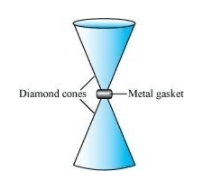Solution. Diameter of the cones at the narrow ends, d=0.50 \mathrm{~mm}=0.5 \times 10^{-3} \mathrm{~m} Radius, r=\frac{d}{2}=0.25 \times 10^{-3} \mathrm{~m} Compressional force, F=50000 \mathrm{~N} Pressure at the tip of the anvil: p=\frac{\text { Force }}{\text { Area\pi }}=\frac{F}{r^{2}} =\frac{50000}{\pi\left(0.25 \times 10^{-3}\right)^{2}} =2.55 \times 10^{11} \mathrm{~Pa} Therefore, the pressure at the tip of the anvil is 2.55 \times 10^{11} \mathrm{~Pa}. Question 18: A rod of length 1.05 \mathrm{~m} having negligible mass is supported at its ends by two wires of steel (wire A) and aluminium (wire B) of equal lengths as shown in Fig. 9.15. The cross-sectional areas of wires \mathrm{A} and \mathbf{B} are 1.0 \mathrm{~mm}^{2} and 2.0 \mathrm{~mm}^{2}, respectively. At what point along the rod should a mass m be suspended in order to produce (a) equal stresses and (b) equal strains in both steel and aluminium wires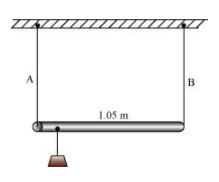Solution. (a) 0.7 m from the steel-wire end (b) 0.432 m from the steel-wire end Cross-sectional area of wire A, a_{1}=1.0 \mathrm{~mm}^{2}=1.0 \times 10^{-6} \mathrm{~m}^{2}Cross-sectional area of wire \mathbf{B}, a_{2}=2.0 \mathrm{~mm}^{2}=2.0 \times 10^{-6} \mathrm{~m}^{2} Young's modulus for steel, Y_{1}=2 \times 10^{11} \mathrm{Nm}^{-2} Young's modulus for aluminium, Y_{2}=7.0 \times 10^{10} \mathrm{Nm}^{-2} (a) Let a small mass m be suspended to the rod at a distance y from the end where wire A is attached. Stress in the wire =\frac{\text { Force }}{\text { Area }}=\frac{F}{a} If the two wires have equal stresses, then: \frac{F_{1}}{a_{1}}=\frac{F_{2}}{a_{2}} If the two wires have equal stresses, then: \frac{F_{1}}{a_{1}}=\frac{F_{2}}{a_{2}} Where, F_{1}= Force exerted on the steel wire F_{2}= Force exerted on the aluminum wire \frac{F_{1}}{F_{2}}=\frac{a_{1}}{a_{2}}=\frac{1}{2}$$\ldots(i)$
The situation is shown in the following figure.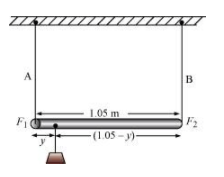Taking torque about the point of suspension, we have:
$F_{1} y=F_{2}(1.05-y)$
$\frac{F_{1}}{F_{2}}=\frac{(1.05-y)}{y}$$\ldots(i i) Using equations (i) and (ii), we can write: \frac{(1.05-y)}{y}=\frac{1}{2} 2(1.05-y)=y 2.1-2 y=y 3 y=2.1 \therefore y=0.7 \mathrm{~m} In order to produce an equal stress in the two wires, the mass should be suspended at a distance of 0.7 m from the end where wire A is attached. (b) Young's modulus =\frac{\text { Stress }}{\text { Strain }} Strain =\frac{\text { Stress }}{\text { Young's modulus }}=\frac{\frac{F}{a}}{Y}’ If the strain in the two wires is equal, then: \frac{F_{1}}{\frac{a_{1}}{Y_{1}}}=\frac{F_{2}}{\frac{a_{2}}{Y_{2}}} \frac{F_{1}}{F_{2}}=\frac{a_{1}}{a_{2}} \frac{Y_{1}}{Y_{2}}=\frac{1}{2} \times \frac{2 \times 10^{11}}{7 \times 10^{10}}=\frac{10}{7}... (iii) Taking torque about the point where mass m, is suspended at a distance y1 from the side where wire A attached, we get: F_{1} y_{1}=F_{2}\left(1.05-y_{1}\right) \frac{F_{1}}{F_{2}}=\frac{\left(1.05-y_{1}\right)}{y_{1}} \ldots (iii) Using equations (iii) and (iv), we get: \frac{\left(1.05-y_{1}\right)}{y_{1}}=\frac{10}{7} 7\left(1.05-y_{1}\right)=10 y_{1} 17 y_{1}=7.35 \therefore y_{1}=0.432 \mathrm{~m} In order to produce an equal strain in the two wires, the mass should be suspended at a distance of 0.432 m from the end where wire A is attached. Question 19: A mild steel wire of length 1.0 \mathrm{~m} and cross-sectional area 0.50 \times 10^{-2} \mathrm{~cm}^{2} is stretched, well within its elastic limit, horizontally between two pillars. A mass of 100 \mathrm{~g} is suspended from the mid-point of the wire. Calculate the depression at the midpoint. Solution.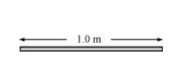Length of the steel wire =1.0 \mathrm{~m} Area of cross-section, A=0.50 \times 10^{-2} \mathrm{~cm}^{2}=0.50 \times 10^{-6} \mathrm{~m}^{2} A mass 100 \mathrm{~g} is suspended from its midpoint. m=100 \mathrm{~g}=0.1 \mathrm{~kg} Hence, the wire dips, as shown in the given figure.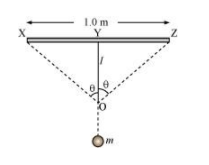Original length =X Z Depression =1 The length after mass m, is attached to the wire =\mathrm{XO}+\mathrm{OZ} Increase in the length of the wire: \Delta l=(\mathrm{XO}+\mathrm{OZ})-\mathrm{XZ} Where, \mathrm{XO}=\mathrm{OZ}=\left[(0.5)^{2}+l^{2}\right]^{\frac{1}{2}} \therefore \Delta l=2\left[(0.5)^{2}+(l)^{2}\right]^{\frac{1}{2}}-1.0 =2 \times 0.5\left[1+\left(\frac{l}{0.5}\right)^{2}\right]^{\frac{1}{2}}-1.0 Expanding and neglecting higher terms, we get: \Delta l=\frac{l^{2}}{0.5} Strain =\frac{\text { Increase in length }}{\text { Original length }} Let T be the tension in the wire. \therefore m g=2 T \cos \theta Using the figure, it can be written as: \cos \theta=\frac{l}{\left((0.5)^{2}+l^{2}\right)^{\frac{1}{2}}} =\frac{l}{(0.5)\left(1+\left(\frac{l}{0.5}\right)^{2}\right)^{\frac{1}{2}}} Expanding the expression and eliminating the higher terms:’ \cos \theta=\frac{l}{(0.5)\left(1+\frac{l^{2}}{2(0.5)^{2}}\right)} \left(1+\frac{l^{2}}{0.5}\right)=1 for small l \therefore \cos \theta=\frac{l}{0.5} \therefore T=\frac{m g}{2\left(\frac{l}{0.5}\right)}=\frac{m g \times 0.5}{2 l}=\frac{m g}{4 l} Stress =\frac{\text { Tension }}{\text { Area }}=\frac{m g}{4 l \times A} Young's modulus =\frac{\text { Stress }}{\text { Strain }} Y=\frac{m g \times 0.5}{4 l \times A \times l^{2}} I=\sqrt{\frac{m g \times 0.5}{4 Y A}} Young's modulus of steel, Y=2 \times 10^{11} \mathrm{~Pa} \therefore l=\sqrt{\frac{0.1 \times 9.8 \times 0.5}{4 \times 2 \times 10^{11} \times 0.50 \times 10^{-6}}}$$$
=0.0106 \mathrm{~m}
Hence, the depression at the midpoint is $0.0106 \mathrm{~m}$.

Question 20: Two strips of metal are riveted together at their ends by four rivets, each of diameter $6.0 \mathrm{~mm}$. What is the maximum tension that can be exerted by the riveted strip if the shearing stress on the rivet is not to exceed $6.9 \times 10^{7} \mathrm{~Pa}$ ? Assume that each rivet is to carry one quarter of the load.

Solution. Diameter of the metal strip, $d=6.0 \mathrm{~mm}=6.0 \times 10^{-3} \mathrm{~m}$
Radius, $r=\frac{d}{2}=3.0 \times 10^{-3} \mathrm{~m}$
Maximum shearing stress $=6.9 \times 10^{7} \mathrm{~Pa}$
Maximum stress $=\frac{\text { Maximum load or force }}{\text { Area }}$
Maximum force $=$ Maximum stress $\times$ Area
$=6.9 \times 10^{7} \times \pi \times(r)^{2}$
$=6.9 \times 10^{7} \times \pi \times\left(3 \times 10^{-3}\right)^{2}$
$=1949.94 \mathrm{~N}$
Each rivet carries one quarter of the load.
$\therefore$ Maximum tension on each rivet $=4 \times 1949.94=7799.76 \mathrm{~N}$

Question 21: The Marina trench is located in the Pacific Ocean, and at one place it is nearly eleven $\mathrm{km}$ beneath the surface of water. The water pressure at the bottom of the trench is about $1.1 \times 10^{8} \mathrm{~Pa}$. A steel ball of initial volume $0.32 \mathrm{~m}^{3}$ is dropped into the ocean and falls to the bottom of the trench. What is the change in the volume of the ball when it reaches to the bottom?

Solution. Water pressure at the bottom, $p=1.1 \times 10^{8} \mathrm{~Pa}$
Initial volume of the steel ball, $V=0.32 \mathrm{~m}^{3}$
Bulk modulus of steel, $B=1.6 \times 10^{11} \mathrm{Nm}^{-2}$
The ball falls at the bottom of the Pacific Ocean, which is $11 \mathrm{~km}$ beneath the surface.
Let the change in the volume of the ball on reaching the bottom of the trench be $\Delta V$.
Bulk modulus, $B=\frac{p}{\frac{\Delta V}{V}}$
$\Delta V=\frac{B}{p V}$
$=\frac{1.1 \times 10^{8} \times 0.32}{1.6 \times 10^{11}}$
$=2.2 \times 10^{-4} \mathrm{~m}^{3}$
Therefore, the change in volume of the ball on reaching the bottom of the trench is $2.2 \times 10^{-4} \mathrm{~m}^{3}$.